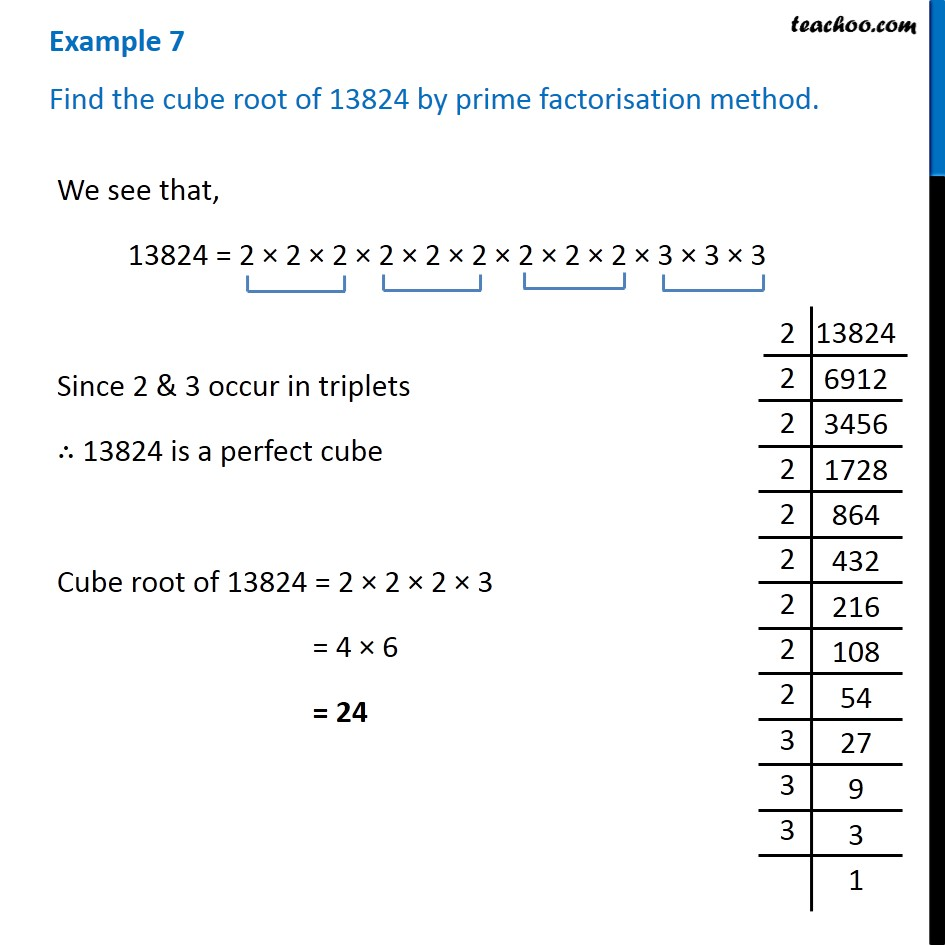Examples

Chapter 6 Class 8 Cubes and Cube Roots
Serial order wiseLearn in your speed, with individual attention - Teachoo Maths 1-on-1 Class

### Transcript

Example 7 Find the cube root of 13824 by prime factorisation method. We see that, 13824 = 2 × 2 × 2 × 2 × 2 × 2 × 2 × 2 × 2 × 3 × 3 × 3 Since 2 & 3 occur in triplets ∴ 13824 is a perfect cube Cube root of 13824 = 2 × 2 × 2 × 3 = 4 × 6 = 24